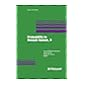Normal view MARC view ISBD view

# Seminar on stochastic processes, 1989. Progress in probability. Vol 18.

Additional authors: Chung, K.L. | Getoor, R.K. | Fitzsimmons, P.J.519.08 Probability in Banach spaces, 9 / 519.08 Encyclopedia of mathematics and its applications. Vol 19. Birkhoff interpolation. 519.08 Encyclopedia of mathematics and its applications. Vol 8. Angular momentum in quantum physics ; theory and application. 519.08 Seminar on stochastic processes, 1989. Progress in probability. Vol 18. 519.08 Sums, trimmed sums and extremes. Progress in probability. Vol 23. 519.08 Seminar on stochastic processes, 1990. Progress in probability. Vol 24. 519.08 Encyclopedia of mathematics and its applications. Vol 10. Product integration with applications to differential equations.